• 三、CRF损失函数 CRF损失函数由两部分组成，真实路径的分数 和 所有路径的总分数。真实路径的分数应该是所有路径中分数最高的。 例如，我们的数据集中有如下几种类别： Label Index B-Person 0 I-...
本文翻译，原文地址：

https://createmomo.github.io/2017/10/08/CRF-Layer-on-the-Top-of-BiLSTM-3/

https://createmomo.github.io/2017/10/17/CRF-Layer-on-the-Top-of-BiLSTM-4/

https://createmomo.github.io/2017/11/11/CRF-Layer-on-the-Top-of-BiLSTM-5/

三、CRF损失函数

CRF损失函数由两部分组成，真实路径的分数 和 所有路径的总分数。真实路径的分数应该是所有路径中分数最高的。

例如，我们的数据集中有如下几种类别：

Label
Index
B-Person
0
I-Person
1
B-Organization
2
I-Organization
3
O
4
START
5
END
6
一个包含5个单词的句子，可能的类别序列如下：

1) START B-Person B-Person B-Person B-Person B-Person END
2) START B-Person I-Person B-Person B-Person B-Person END
…
10) START B-Person I-Person O B-Organization O END
…
N) O O O O O O O
假设每种可能的路径的分数为，共有条路径，则路径的总分是:

，是一个数学常量。（下节会详细介绍如何计算，这里你可以把它当作这条路径的分数）。

如果第十条路径是真实路径，也就是说第十条是正确预测结果，那么应该是所有可能路径里分数最高的。根据如下损失函数，在训练过程中，BiLSTM-CRF模型的参数值将随着训练过程的迭代不断更新，使得真实路径所占的比值越来越大。

那么现在的问题就是：

如何定义路径的分数
怎么计算所有路径的分数
当计算所有的路径分时，是否需要穷举多有可能的路径？（答案是不需要）
四、真实路径分数

很明显，在所有的可能的路径中有一条是真实的路径。比如在上述的类别序列中，“START B-Person I-Person O B-Organization O END”是一条真实的路径。不正确的路径如“START B-Person B-Organization O I-Person I-Person B-Person”。是第条路径的分数。

在训练阶段，crf的损失函数只需要两种类别的分数，真实路径的分数 和 所有路径的总分数。在所有的可能路径的分数中，真实路径的分数应该是最高的。

当然计算是十分简单的，下面让我们聚焦如何来计算。

我们假设“START B-Person I-Person O B-Organization O END”是一条真实的路径：

一个句子中有5个单词，分别是
添加开始和结尾单词，分别是
包含两个部分，
tips:Emission Score 和 Transition Score在《BiLSTM中的CRF层（二）CRF层》一文中已经介绍。

（1）Emission Score（发射分数）:

是第索引的单词被标注为的分数
这些分数来自前一层BiLstm的输出
可以设置为0
（2）Transition Score（转移分数）:

是从label1转移到label2的分数
这些分数来自于CRF层。换句话说，这些分数其实是CRF层的参数
五、所有路径的分数

上面提到所有路径的分数。计算所有路径的总分最简单的方式是：穷举所有可能的路径，分别计算路径分，最后求和。但是这种方式是十分低效的，而且训练时间也是不可忍受的。

下面以一个简单的例子来说明如何高效的计算所有路径的分数。

Step1： recall the CRF loss function

CRF损失函数的定义是，我们把它变成log损失函数如下：

，由于我们训练的目标通常是最小化损失函数，所以我们加上负号：

（原文中在求真实路径的分数的时候，求和用的是N和N-1，但是从上面的求解发射分数和转移分数公式看，这里的N应该是句子的长度words，N-1应该是标签数num_tag，所有的路径总数N应该是N=(num_tag)^(words)，否则对应不上。不知道是不是作者为了简化统一用N表示，还是本人理解错误。。。囧囧囧）

在上面，我们已经知道如何计算真实路径的分数，下面我们只需要找到一种高效的方式来计算。

Step2：recall the Emission and Transition Score

为了简化说明，这里我们假设我们训练模型的句子只有3的长度，标签只有2种：

我们从Bi-Lstm层获得的发射分数如下：

tips:表示单词被标签为的分数。

从CRF层获得的转移分数如下：

tips:表示从标签转移到标签的分数

Step3：START FIGHTING!

首先要记得我们的目标是

整个过程其实是分数累加的过程。它的思想和动态规划如出一辙。总而言之就是，首先单词的所有路径的总分被计算出来；然后，计算从 的所有路径的得分，最后计算的所有路径的得分，也就是我们最终要的结果。

接下来，你会看到两个变量：obs和 previous。previous存储了之前步骤的结果，obs代表当前单词的信息。

=======================================================================================================

：

如果我们的句子只有一个单词，前一个步骤没有任何的结果，因此previous=None。另外，我们只能获取到，和是上面提到的从BiLSTM层获得的发射分数。

因此，这里单词的所有路径分数为：

=======================================================================================================

：

(1) 将previous扩展为：

(2) 将obs扩展为：

将previos和obs扩展是为了计算总分更加高效。下面将会看到

(3) 对previou、obs和转移分数求和：

然后改变previos的值

到这里，第二次迭代实际上已经完成了。然后，我们使用新的previous值来计算的所有路径总分：

从上面公式可以看出，这就是我们的求解目标

在上述公式中：

=======================================================================================================

下面的手码太累了，直接贴原文的截图，截图出自（https://createmomo.github.io/2017/11/11/CRF-Layer-on-the-Top-of-BiLSTM-5/），原理是一样的。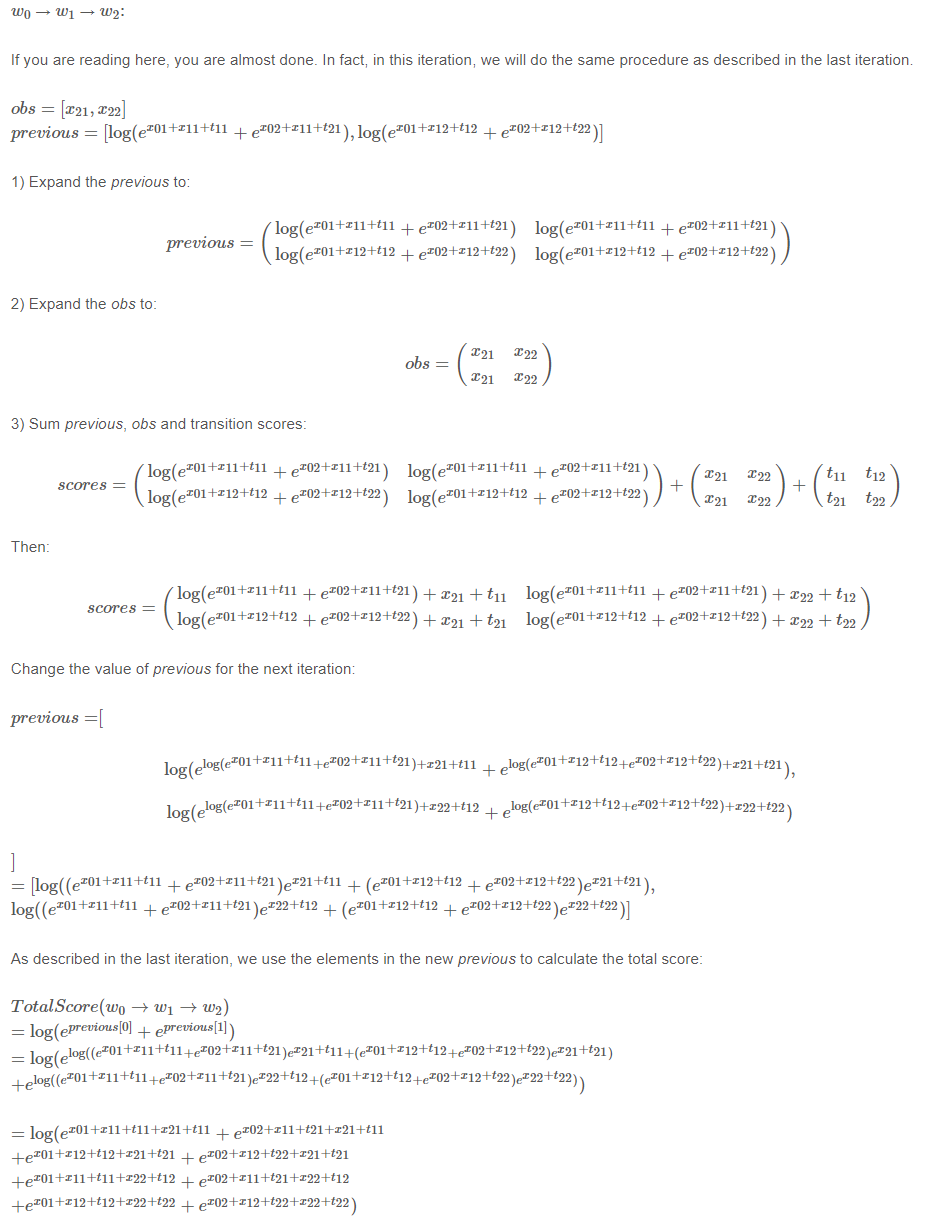=======================================================================================================

上述过程建议用手推一遍，对理解这个过程十分有用。。。

至此，我们完成了的求解过程。

六、如何利用CRF来推理

前面几节介绍了Bi-Lstm-CRF的模型结构以及CRF损失函数。我们可以使用开源的深度学习框架（Keras、tensorflow等）来实现一个Bi-Lstm-CRF模型。而且用这些框架最好的是不用自己来实现反向传播这个过程，更有的框架已经实现了CRF层，只需要添加一行代码就可以完全实现Bi-Lstm-CRF模型。

推理的过程和上述求解所有路径总分的过程有点相似，每次获取得分最高的路径，最终选择所有路径中得分最高的路径为最佳路径。

详细过程见原文：https://createmomo.github.io/2017/11/24/CRF-Layer-on-the-Top-of-BiLSTM-6/

这里不再讲述，因为整个过程十分简单，理解了第五节中的求解过程即可。

下一篇：

下一篇将会介绍如何利用Tensorflow1.x版本来实现一个Bi-Lstm-CRF模型

参考文档：

 Lample, G., Ballesteros, M., Subramanian, S., Kawakami, K. and Dyer, C., 2016. Neural architectures for named entity recognition. arXiv preprint arXiv:1603.01360.

展开全文• 非常感谢啦，我找了很久都没有得到答案，可能是缺乏经验。。。。。。。。。。。谢谢谢谢谢谢开发语言
• 上图是1000个样本训练模型的损失函数（mape 平均绝对百分误差）结果，下图为样本数量增加到2000个，损失函数就看不懂了，每个epoch下降到80左右就上跳到一个较大的值，尤其是当mape在80多的时候accracy都为0。...tensorflow 深度学习
• CRF层2.1 Emission得分2.2 Transition得分2.3 CRF损失函数2.4 实际路径得分2.5 所有可能的路径的得分附言 转载来源：CreateMoMo 今天是第二部分，给大家推导一下CRF的损失函数如何计算，思路很清楚。 回顾 在前...
文章目录回顾2. CRF层2.1 Emission得分2.2 Transition得分2.3 CRF损失函数2.4 实际路径得分2.5 所有可能的路径的得分附言
转载来源：CreateMoMo

今天是第二部分，给大家推导一下CRF的损失函数如何计算，思路很清楚。

回顾
在前一节中，我们知道CRF层可以从训练数据集中学习一些约束，以确保最终预测的实体标签序列是有效的。
约束条件可以是:

句子中第一个单词的标签应该以“B-”或“O”开头，而不是“I-”
“B-label1 I-label2 I-label3 I-…”，在这个模式中，label1、label2、label3…应该是相同的命名实体标签。例如，“B-Person I-Person”是有效的，但是“B-Person I-Organization”是无效的。
“O I-label”无效。一个命名实体的第一个标签应该以“B-”而不是“I-”开头，换句话说，有效的模式应该是“O B-label”
…
阅读本文之后，你将了解为什么CRF层可以学习这些约束。

2. CRF层
在CRF层的损失函数中，我们有两种类型的分数。这两个分数是CRF层的关键概念。
2.1 Emission得分
第一个是emission分数。这些emission分数来自BiLSTM层。例如，如图2.1所示，标记为B-Person的w0的分数为1.5。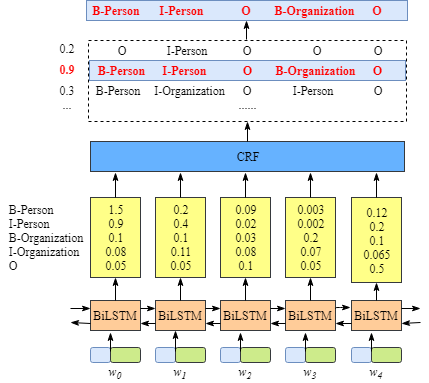为了方便起见，我们将给每个标签一个索引号，如下表所示。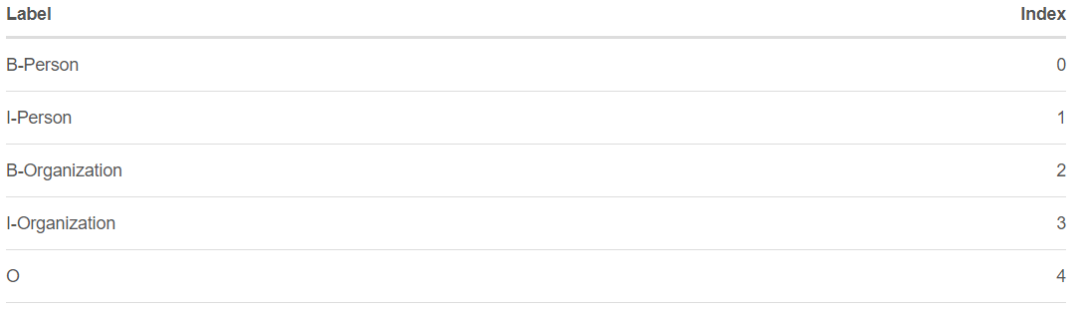我们用$x_{iy_{f}}$来表示emission分数。i是word的索引，$y_{i}$是label的索引。如图2.1所示，$x_{i=1,y_{i}=2}=x_{w_{1},B-Organization}=0.1,=1$，即w1作为B-Organization的得分为0.1。
2.2 Transition得分
我们使用$t_{y_{i},y_{j}}$来表示transition分数。例如，$t_{B-Person,I-Person}=0.9$表示标签的transition，$B-Person\rightarrow I-Person$得分为0.9。因此，我们有一个transition得分矩阵，它存储了所有标签之间的所有得分。
为了使transition评分矩阵更健壮，我们将添加另外两个标签，START和END。START是指一个句子的开头，而不是第一个单词。END表示句子的结尾。
下面是一个transition得分矩阵的例子，包括额外添加的START和END标签。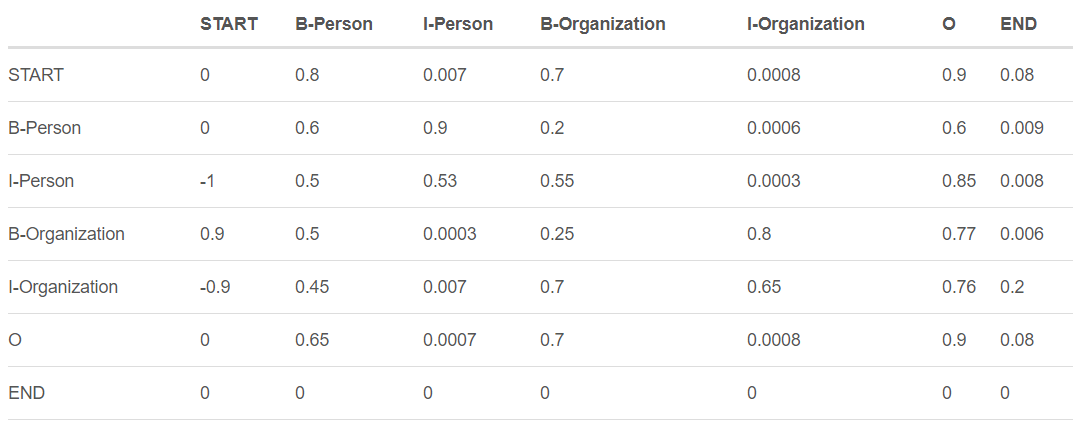如上表所示，我们可以发现transition矩阵已经学习了一些有用的约束。

句子中第一个单词的标签应该以“B-”或“O”开头，而不是“I-”开头**(从“START”到“I- person或I- organization”的transition分数非常低)**
“B-label1 I-label2 I-label3 I-…”，在这个模式中，label1、label2、label3…应该是相同的命名实体标签。例如，“B-Person I-Person”是有效的，但是“B-Person I-Organization”是无效的。(例如，从“B-Organization”到“I-Person”的分数只有0.0003，比其他分数低很多)
“O I-label”无效。一个被命名实体的第一个标签应该以“B-”而不是“I-”开头，换句话说，有效的模式应该是“O B-label”(同样，$t_{O,I-Person}$的分数非常小)
…
你可能想问一个关于矩阵的问题。在哪里或如何得到transition矩阵？

实际上，该矩阵是BiLSTM-CRF模型的一个参数。在训练模型之前，可以随机初始化矩阵中的所有transition分数。所有的随机分数将在你的训练过程中自动更新。换句话说，CRF层可以自己学习这些约束。我们不需要手动构建矩阵。随着训练迭代次数的增加，分数会逐渐趋于合理。
2.3 CRF损失函数
CRF损失函数由真实路径得分和所有可能路径的总得分组成。在所有可能的路径中，真实路径的得分应该是最高的。
例如，如果我们的数据集中有如下表所示的这些标签：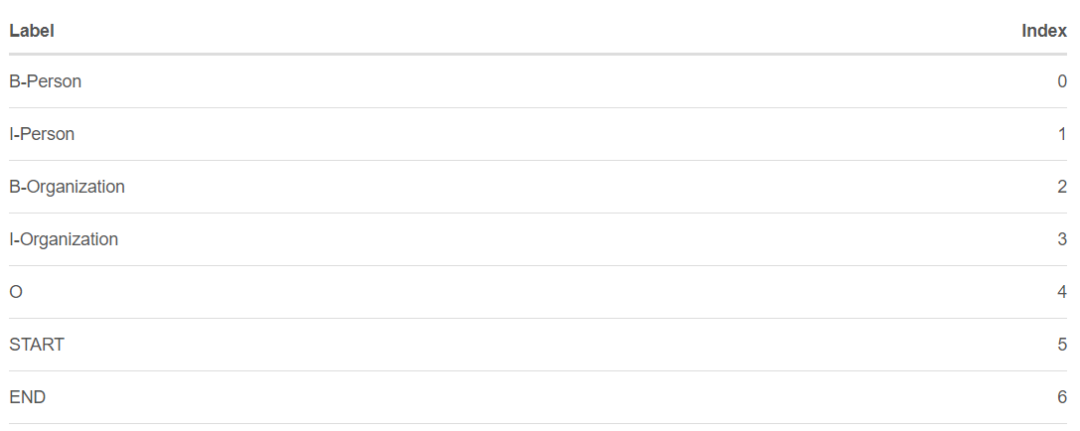我们还是有一个5个单词的句子。可能的路径是：

START B-Person B-Person B-Person B-Person B-Person END

START B-Person I-Person B-Person B-Person B-Person END
…

START B-Person I-Person O B-Organization O END

…
N) O O O O O O O
假设每条可能的路径都有一个分数$P_{i}$，并且总共有N条可能的路径，所有路径的总分数是$P_{total}=P_{1}+P_{2}+...+P_{N}=e^{S_{1}}+e^{S_{2}}+...+e^{S_{N}}$。(在第2.4节中，我们将解释如何计算$S_{i}$，你也可以把它当作这条路径的分数。)

如果我们说第10条路径是真正的路径，换句话说，第10条路径是我们的训练数据集提供的黄金标准标签。在所有可能的路径中，得分$P_{10}$应该是百分比最大的。
在训练过程中，我们的BiLSTM-CRF模型的参数值将会一次又一次的更新，以保持增加真实路径的分数百分比。
$LossFunction=\frac {P_{RealPath}}{P_{1}+P_{2}+...+P_{N}}$
现在的问题是：1)如何定义一个路径的分数？2)如何计算所有可能路径的总分？3)当我们计算总分时，我们需要列出所有可能的路径吗？(这个问题的答案是否定的。)
在下面的小节中，我们将看到如何解决这些问题。
2.4 实际路径得分
在2.3节中，我们假设每条可能的路径都有一个得分$P_{i}$，并且有N条可能的路径，所有路径的总得分为$P_{total}=P_{1}+P_{2}+...+P_{N}=e^{S_{1}}+e^{S_{2}}+...+e^{S_{N}}$。
显然，在所有可能的路径中，一定有一条是真实路径。对于这个例子来说，第1.2节中句子的实际路径是**“START B-Person I-Person O B-Organization O END”**。其他的是不正确的，如“START B-Person B-Organization O I-Person I-Person B-Person”。$e^{S_{i}}$是第i条路径的得分。
在训练过程中，CRF损失函数只需要两个分数：真实路径的分数和所有可能路径的总分数。所有可能路径的分数中，真实路径分数所占的比例会逐渐增加。
计算实际路径分数$e^{S_{i}}$非常简单。
这里我们主要关注的是$S_{i}$的计算。
选取真实路径，“START B-Person I-Person O B-Organization O END”，我们以前用过，例如：

我们有一个5个单词的句子，w1,w2,w3,w4, w4,w5
我们增加了两个额外的单词来表示一个句子的开始和结束，w0,w6
$S_{i}$由两部分组成$S_{i}=EmissionScore+TransitionScore$

Emission得分：
$EmissionScore=x_{0,START}+x_{1,B-Person}+x_{2,I-Person}+x_{3,O}+x_{4,B-Organization}+x_{5,O}+x_{6,END}$

$x_{index,label}$是第index个单词被label标记的分数
这些得分$x_{1,B-Person}+x_{2,I-Person}+x_{3,O}+x_{4,B-Organization}+x_{5,O}$来自之前的BiLSTM输出。
对于$x_{0,START}$，$x-{6,END}$，我们可以把它们设为0。

Transition得分：
$\text {EmissionScore}=x_{0, S T A R T}+x_{1, B-P e r s o n}+x_{2, I-P e r s o n}+x_{3, O}+x_{4, B-O r g a n i z a t i o n}+x_{5, O}+x_{6, E N D}$

$t_{l a b e l 1->l a b e l 2}$是从 label1到label2的transition分数
这些分数来自CRF层。换句话说，这些transition分数实际上是CRF层的参数。
综上所述，现在我们可以计算出$S_{i}$以及路径得分$e^{S_{i}}$。

下一步是如何计算所有可能路径的总分？
2.5 所有可能的路径的得分
如何逐步计算一个toy例子一个句子的所有可能的路径的总分。
在上一节中，我们学习了如何计算一个路径(即$e^{S_{i}}$)的标签路径得分。到目前为止，我们还有一个需要解决的问题，就是如何得到所有路径的总分($P_{\text {total}}=P_{1}+P_{2}+\ldots+P_{N}=e^{S_{1}}+e^{S_{2}}+\ldots+e^{S_{N}}$)。
衡量总分最简单的方法是：列举所有可能的路径并将它们的分数相加。是的，你可以用这种方法计算总分。然而，这是非常低效的。训练的时间将是难以忍受的。
在探索以下内容之前，我建议你先准备一张白纸和一支笔，并按照示例中列出的步骤进行操作。我相信这将有助于你更好地理解算法的细节。此外，你应该知道如何用你喜欢的编程语言实现它。
步骤1: 回想一下CRF损失函数
在section 2.3中，我们将CRF损失函数定义为：
$\text {LossFunction}=\frac{P_{\text {RealPath}}}{P_{1}+P_{2}+\ldots+P_{N}}$
现在我们把loss函数变成log loss函数：
$\text {logLossFunction}=\log \frac{P_{\text {RealPath}}}{P_{1}+P_{2}+\ldots+P_{N}}$
因为当我们训练一个模型时，通常我们的目标是最小化我们的损失函数，我们加上一个负号：
$\text {logLossFunction}=-\log \frac{P_{\text {RealPath}}}{P_{1}+P_{2}+\ldots+P_{N}}=-\log \frac{e^{S_{\text {RealPath}}}}{e^{S_{1}}+e^{S_{2}}+\ldots+e^{S_{N}}}=-\left(\log \left(e^{S_{\text {Real }} P_{\text {ath }}}\right)-\log \left(e^{S_{1}}+e^{S_{2}}+\ldots+e^{S_{N}}\right)\right)=-\left(S_{\text {RealPath }}-\log \left(e^{S_{1}}+e^{S_{2}}+\ldots+e^{S_{N}}\right)\right)=-\left(\sum_{i=1}^{N} x_{i y_{j}}+\sum_{i=1}^{N-1} t_{y_{i} y_{i+1}}-\log \left(e^{S_{1}}+e^{S_{2}}+\ldots+e^{S_{N}}\right)\right)$
在上一节中，我们已经知道如何计算实际路径得分，现在我们需要找到一个有效的解决方案来计算$\log \left(e^{S_{1}}+e^{S_{2}}+\ldots+e^{S_{N}}\right)$。
步骤2: 回忆一下Emission和Transition得分
为了简化，我们假设我们从这个句子中训练我们的模型，它的长度只有3：
$x=\left[w_{0}, w_{1}, w_{2}\right]$
此外，在我们的数据集中，我们有两个标签：
$LabelSet =\{l 1, l 2\}$
我们还有Bi-LSTM层输出的Emission分数：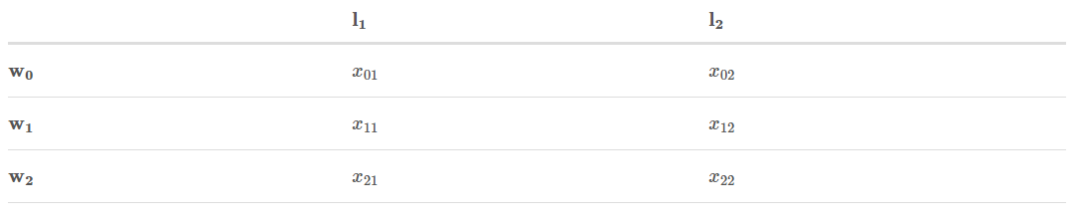$x_{i j}$表示$\boldsymbol{w}_{i}$被标记为$l_{j}$的得分。
此外，Transition分数来自CRF层：
[外链图片转存失败,源站可能有防盗链机制,建议将图片保存下来直接上传(img-reqlK9Na-1591628890932)(CRF Layer on the Top of BiLSTM - 2.assets/1585055587948.png)]
$t_{i} j$是从标签i到标签j的transition得分。
步骤3: 开始战斗（准备好纸笔）
记住：我们的目标是：$\log \left(e^{S_{1}}+e^{S_{2}}+\ldots+e^{S_{N}}\right)$
这个过程就是分数的累加。其思想与动态规划相似(如果你不知道什么是动态编程，也可以继续阅读本文)。我将逐步解释这个例子。但我强烈建议你学习动态规划算法。简而言之，计算w0的所有可能路径的总分。然后，我们用总分来计算w0→w1。最后，我们使用最新的总分来计算w0→w1→w2。我们需要的是最后的总分。
在接下来的步骤中，你将看到两个变量：obs和previous。previous存储前面步骤的最终结果。obs表示当前单词的信息。
$\begin{array}{l} w_{0}: \\ o b s=\left[x_{01}, x_{02}\right] \\ \text { previous }=\text { None } \end{array}$
如果我们的句子只有一个单词$w_{0}$，我们就没有前面步骤的结果，因此previous是None。另外，我们只能观察到第一个词$o b s=\left[x_{01}, x_{02}\right]$。$x_{01}$和$x_{02}$是上述的Emission分数。
你可能会想，的所有可能路径的总分是多少？答案很简单：
$\text {TotalScore}\left(w_{0}\right)=\log \left(e^{x_{01}}+e^{x_{02}}\right)$
$\begin{array}{l} w_{0}->w_{1} \\ o b s=\left[x_{11}, x_{12}\right] \\ \text { previous }=\left[x_{01}, x_{02}\right] \end{array}$

把previous展开成：
$\text {previous}=\left(\begin{array}{ll} x_{01} & x_{01} \\ x_{02} & x_{02} \end{array}\right)$
把obs展开成：
$o b s=\left(\begin{array}{ll} x_{11} & x_{12} \\ x_{11} & x_{12} \end{array}\right)$

你可能想知道，为什么我们需要把previous和obs扩展成矩阵。因为矩阵可以提高计算的效率。在下面的过程中，你将很快看到这一点。

对 previous, obs以及transition得分求和：
$\text {scores}=\left(\begin{array}{cc} x_{01} & x_{01} \\ x_{02} & x_{02} \end{array}\right)+\left(\begin{array}{cc} x_{11} & x_{12} \\ x_{11} & x_{12} \end{array}\right)+\left(\begin{array}{cc} t_{11} & t_{12} \\ t_{21} & t_{22} \end{array}\right)$

然后：
$\text {scores}=\left(\begin{array}{ll} x_{01}+x_{11}+t_{11} & x_{01}+x_{12}+t_{12} \\ x_{02}+x_{11}+t_{21} & x_{02}+x_{12}+t_{22} \end{array}\right)$
为下一个迭代修改previous的值：
$\text {previous}=\left[\log \left(e^{x_{01}+x_{11}+t_{11}}+e^{x_{02}+x_{11}+t_{21}}\right), \log \left(\left(e^{x_{01}+x_{12}+t_{12}}+e^{x_{02}+x_{12}+t_{22}}\right)\right]\right.$
实际上，第二次迭代已经完成。如果有人想知道如何计算所有可能路径的总分(label1→label1, label1→label2, label2→label1, label2→label2)，从w0到w1，可以做如下计算。
我们使用新的previous中的元素：
$\text {TotalScore}\left(w_{0}->w_{1}\right)=\log \left(e^{\text {previous}}+e^{\text {previous}}\right)=\log \left(e^{\log \left(e^{x_{01}+x_{11}+t_{11}}+e^{x_{02}+x_{11}+t_{21}}\right)}+\right.e^{\log \left(e^{\left.x_{01}+x_{12}+t_{12}+e^{x_{02}+x_{12}+t_{22}}\right)}\right)}=\log \left(e^{x_{01}+x_{11}+t_{11}}+e^{x_{02}+x_{11}+t_{21}}+e^{x_{01}+x_{12}+t_{12}}+e^{x_{02}+x_{12}+t_{22}}\right)$
你发现了吗？这正是我们的目标：$\log \left(e^{S_{1}}+e^{S_{2}}+\ldots+e^{S_{N}}\right)$
在这个等式中，我们可以看到：

$S_{1}=x_{01}+x_{11}+t_{11}\left(\text {label}_{1}->\text {label}_{1}\right)$
$S_{2}=x_{02}+x_{11}+t_{21}\left(\operatorname{label}_{2}->\operatorname{label}_{1}\right)$
$S_{3}=x_{01}+x_{12}+t_{12}\left(\operatorname{label}_{1}->\operatorname{label}_{2}\right)$
$S_{4}=x_{02}+x_{12}+t_{22}\left(\text {label}_{2}->\text {label}_{2}\right)$

$w_{0}->w_{1}->w_{2}$
如果你读到这里了，你已经快要读完了，实际上，在这个迭代里，我们做的事情是和上个迭代一样的。
$\begin{array}{l} \text { obs }=\left[x_{21}, x_{22}\right] \\ \text { previous }=\left[\log \left(e^{x_{01}+x_{11}+t_{11}}+e^{x_{02}+x_{11}+t_{21}}\right), \log \left(\left(e^{x_{01}+x_{12}+t_{12}}+e^{x_{02}+x_{12}+t_{22}}\right)\right]\right. \end{array}$

把previous扩展成：
$\text {previous}=\left(\begin{array}{cc} \log \left(e^{x_{01}+x_{11}+t_{11}}+e^{x_{02}+x_{11}+t_{21}}\right) & \log \left(e^{x_{01}+x_{11}+t_{11}}+e^{x_{02}+x_{11}+t_{21}}\right) \\ \log \left(\left(e^{x_{01}+x_{12}+t_{12}}+e^{x_{02}+x_{12}+t_{22}}\right)\right. & \log \left(\left(e^{x_{01}+x_{12}+t_{12}}+e^{x_{02}+x_{12}+t_{22}}\right)\right. \end{array}\right)$
把obs扩展成：
$o b s=\left(\begin{array}{ll} x_{21} & x_{22} \\ x_{21} & x_{22} \end{array}\right)$
把previous, obs和transition分数加起来：
$\text {scores}=\left(\begin{array}{cc} \log \left(e^{x_{01}+x_{11}+t_{11}}+e^{x_{02}+x_{11}+t_{21}}\right) & \log \left(e^{x_{01}+x_{11}+t_{11}}+e^{x_{02}+x_{11}+t_{21}}\right) \\ \log \left(\left(e^{x_{01}+x_{12}+t_{12}}+e^{x_{02}+x_{12}+t_{22}}\right)\right. & \log \left(\left(e^{x_{01}+x_{12}+t_{12}}+e^{x_{02}+x_{12}+t_{22}}\right)\right. \end{array}\right)+\left(\begin{array}{cc} x_{21} & x_{22} \\ x_{21} & x_{22} \end{array}\right)+\left(\begin{array}{ll}t_{11} & t_{12} \\ t_{21} & t_{22}\end{array}\right)$
然后：
$\text {scores}=\left(\begin{array}{cc} \log \left(e^{x_{01}+x_{11}+t_{11}}+e^{x_{02}+x_{11}+t_{21}}\right)+x_{21}+t_{11} & \log \left(e^{x_{01}+x_{11}+t_{11}}+e^{x_{02}+x_{11}+t_{21}}\right)+x_{22}+t_{12} \\ \log \left(\left(e^{x_{01}+x_{12}+t_{12}}+e^{x_{02}+x_{12}+t_{22}}\right)+x_{21}+t_{21}\right. & \log \left(e^{x_{01}+x_{11}+t_{11}}+e^{x_{02}+x_{11}+t_{21}}\right)+x_{22}+t_{22} \end{array}\right)$
为下一个迭代计算 previous的值：
\begin{aligned} &\begin{array}{l} \text { previous }=[ \\ \qquad \begin{array}{l} \log \left(e^{\log \left(e^{\left.x_{01}+x_{11}+t_{11}+e^{x_{02}+x_{11}+t_{21}}\right)+x_{21}+t_{11}}+e^{\log \left(e^{\left.x_{01}+x_{12}+t_{12}+e^{x_{02}+x_{12}+t_{22}}\right)+x_{21}+t_{21}}\right)}\right.}\right. \\,\\ \log \left(e^{\log \left(e^{\left.x_{01}+x_{11}+t_{11}+e^{x_{02}+x_{11}+t_{21}}\right)+x_{22}+t_{12}}+e^{\log \left(e^{\left.x_{01}+x_{12}+t_{12}+e^{x_{02}+x_{12}+t_{22}}\right)+x_{22}+t_{22}}\right)}\right.}\right. \end{array} \end{array}\\ ]\\ &=\left[\log \left(\left(e^{x_{01}+x_{11}+t_{11}}+e^{x_{02}+x_{11}+t_{21}}\right) e^{x_{21}+t_{11}}+\left(e^{x_{01}+x_{12}+t_{12}}+e^{x_{02}+x_{12}+t_{22}}\right) e^{x_{21}+t_{21}}\right)\right.\\ &\left.\log \left(\left(e^{x_{01}+x_{11}+t_{11}}+e^{x_{02}+x_{11}+t_{21}}\right) e^{x_{22}+t_{12}}+\left(e^{x_{01}+x_{12}+t_{12}}+e^{x_{02}+x_{12}+t_{22}}\right) e^{x_{22}+t_{22}}\right)\right] \end{aligned}
就像下一个迭代描述的一样，我们使用新的previous中的元素来计算total score：
$\begin{array}{l} \text { TotalScore }\left(w_{0} \rightarrow w_{1} \rightarrow w_{2}\right) \\ =\log \left(e^{\text {previous}}+e^{\text {previous}}\right) \\ =\log \left(e^{\log \left(\left(e^{\left.x_{01}+x_{11}+t_{11}+e^{x_{02}+x_{11}+t_{21}}\right) e^{x_{21}+t_{11}}+\left(e^{\left.\left.x_{01}+x_{12}+t_{12}+e^{x_{02}+x_{12}+t_{22}}\right) e^{x_{21}+t_{21}}\right)}\right.}\right.\right.}\right. \\ +e^{\log \left(\left(e^{\left.x_{01}+x_{11}+t_{11}+e^{x_{02}+x_{11}+t_{21}}\right) e^{x_{22}+t_{12}+\left(e^{\left.\left.x_{01}+x_{12}+t_{12}+e^{x_{02}+x_{12}+t_{22}}\right) e^{x_{22}+t_{22}}\right)}\right)}}\right.\right.} \\ =\log \left(e^{x_{01}+x_{11}+t_{11}+x_{21}+t_{11}}+e^{x_{02}+x_{11}+t_{21}+x_{21}+t_{11}}\right. \\ +e^{x_{01}+x_{12}+t_{12}+x_{21}+t_{21}}+e^{x_{02}+x_{12}+t_{22}+x_{21}+t_{21}} \\ +e^{x_{01}+x_{11}+t_{11}+x_{22}+t_{12}}+e^{x_{02}+x_{11}+t_{21}+x_{22}+t_{12}} \\ \left.+e^{x_{01}+x_{12}+t_{12}+x_{22}+t_{22}}+e^{x_{02}+x_{12}+t_{22}+x_{22}+t_{22}}\right) \end{array}$

恭喜！
我们达到了目标，$\log \left(e^{S_{1}}+e^{S_{2}}+\ldots+e^{S_{N}}\right)$，我们的toy句子有三个单词，label set有两个label，所以一共应该有8种可能的label path。
附言
在你享用一杯咖啡或一块甜蛋糕休息之前，请允许我说几句话。虽然你发现这个过程相当复杂，但是实现这个算法要容易得多。使用计算机的优点之一是可以完成一些重复性的工作。现在你可以自己实现CRF损失函数，并开始训练自己的模型。

英文原文：https://createmomo.github.io/2017/09/23/CRF_Layer_on_the_Top_of_BiLSTM_2


展开全文• 这里写自定义目录标题关于LSTM两个激活函数的问题 关于LSTM两个激活函数的问题 LSTM中有两个激活函数：activation 和recurrent_activation ...----如果两个激活函数相同，结果loss出现nan（楼主损失函数为mse） 注意
这里写自定义目录标题关于LSTM两个激活函数的问题
关于LSTM两个激活函数的问题
LSTM中有两个激活函数：activation 和recurrent_activation
recurrent_activation是针对三个门机制（遗忘门、输入门、输出门）的激活函数，而activation是针对输入信息（默认tanh）和当前隐状态输出（默认tanh）的激活函数。理论上，两个激活函数都可以修改的。但是，有一点很有趣的现象
----如果两个激活函数相同，结果loss出现nan（楼主损失函数为mse）
注意，如果是可能出现，我就不写这篇了。主要是，必然出现。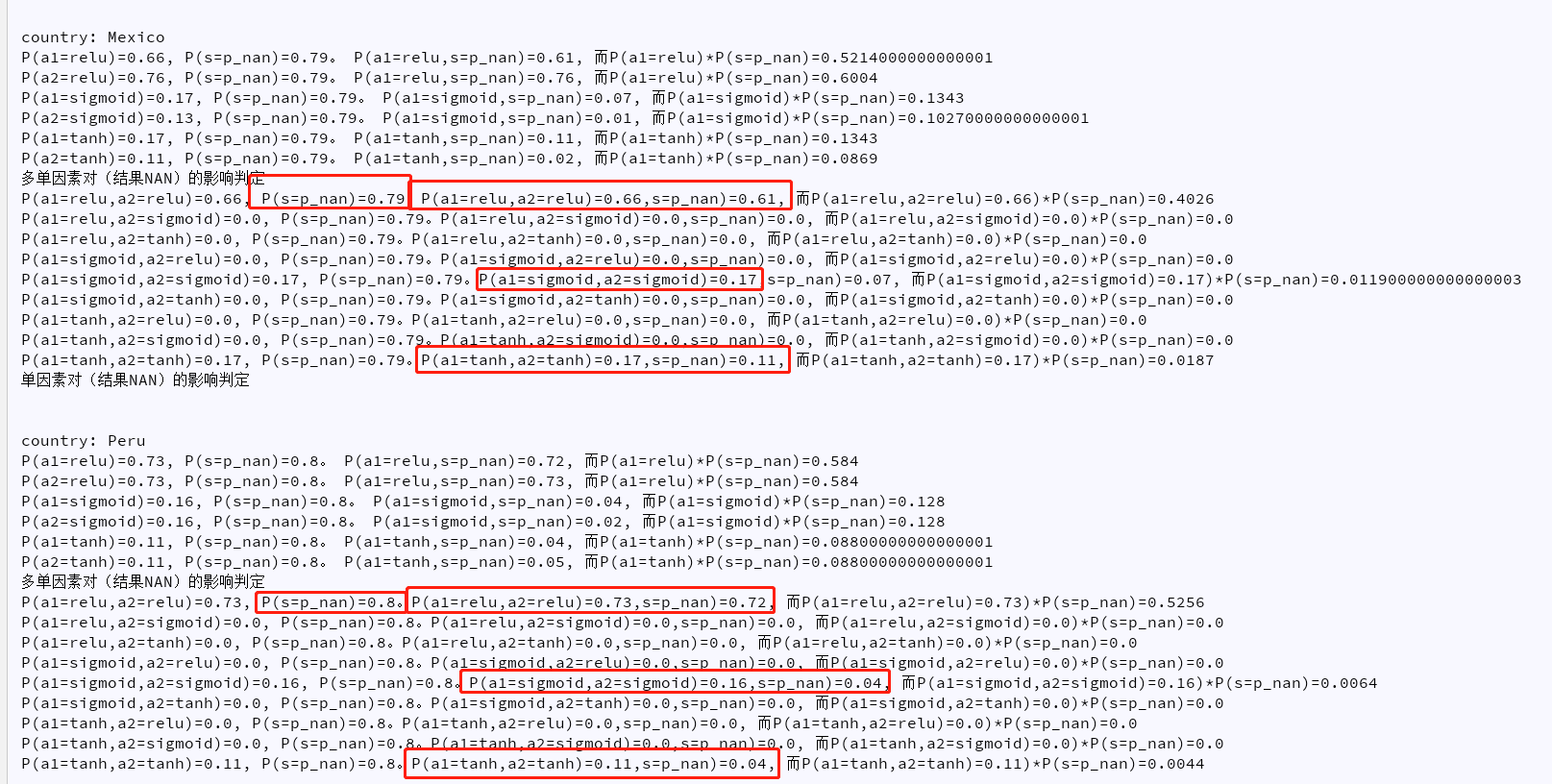以上是部分统计结果截图。楼主做的是探索不同国家的新冠数据使用LSTM的超参数及预测情况。结果出现了一大堆NAN。在超参数组合不多的时候，还以为是随机性出现NAN。后面组合增加到100个之后，对不同国家分别跑了以下，结果发现NAN实在太多。于是准备用条件概率计算独立性，结果发现不需要条件概率，联合概率就可以发现了。
结论：
P(a1=a2，s=nan) = P(s=nan) = P(a1=a2)
==》
P(a1=a2 | s=nan)
= P(s=nan | a1=a2 )
= P(a1=a2，s=nan)/P(s=nan)
=P(a1=a2，s=nan)/P(a1=a2)
=1

a1：activation,
a2:recurrent_activation,
s:loss mse

楼主水平有限，如果是因为很简单的原因，欢迎大佬来diss，顺便给我科普一波。
如果楼主后面的研究中发现原因，会再修改帖子的。


展开全文深度学习 tensorflow 神经网络 机器学习
• 作者：CreateMoMo编译：ronghuaiyang导读今天是第二部分，给大家推导一下CRF的损失函数如何计算，思路很清楚。回顾在前一节中，我们知道CRF层可以从训练数据集中学习一些...


作者：CreateMoMo编译：ronghuaiyang导读今天是第二部分，给大家推导一下CRF的损失函数如何计算，思路很清楚。回顾在前一节中，我们知道CRF层可以从训练数据集中学习一些约束，以确保最终预测的实体标签序列是有效的。约束条件可以是:句子中第一个单词的标签应该以“B-”或“O”开头，而不是“I-”“B-label1 I-label2 I-label3 I-…”，在这个模式中，label1、label2、label3…应该是相同的命名实体标签。例如，“B-Person I-Person”是有效的，但是“B-Person I-Organization”是无效的。“O I-label”无效。一个命名实体的第一个标签应该以“B-”而不是“I-”开头，换句话说，有效的模式应该是“O B-label”…阅读本文之后，你将了解为什么CRF层可以学习这些约束。2. CRF层在CRF层的损失函数中，我们有两种类型的分数。这两个分数是CRF层的关键概念。2.1 Emission得分第一个是emission分数。这些emission分数来自BiLSTM层。例如，如图2.1所示，标记为B-Person的w0的分数为1.5。为了方便起见，我们将给每个标签一个索引号，如下表所示。我们用来表示emission分数。i是word的索引，是label的索引。如图2.1所示，=1，即w1作为B-Organization的得分为0.1。2.2 Transition得分我们使用来表示transition分数。例如，表示标签的transition，得分为0.9。因此，我们有一个transition得分矩阵，它存储了所有标签之间的所有得分。为了使transition评分矩阵更健壮，我们将添加另外两个标签，START和END。START是指一个句子的开头，而不是第一个单词。END表示句子的结尾。下面是一个transition得分矩阵的例子，包括额外添加的START和END标签。如上表所示，我们可以发现transition矩阵已经学习了一些有用的约束。句子中第一个单词的标签应该以“B-”或“O”开头，而不是“I-”开头**(从“START”到“I- person或I- organization”的transition分数非常低)**“B-label1 I-label2 I-label3 I-…”，在这个模式中，label1、label2、label3…应该是相同的命名实体标签。例如，“B-Person I-Person”是有效的，但是“B-Person I-Organization”是无效的。(例如，从“B-Organization”到“I-Person”的分数只有0.0003，比其他分数低很多)“O I-label”无效。一个被命名实体的第一个标签应该以“B-”而不是“I-”开头，换句话说，有效的模式应该是“O B-label”(同样，的分数非常小)…你可能想问一个关于矩阵的问题。在哪里或如何得到transition矩阵？实际上，该矩阵是BiLSTM-CRF模型的一个参数。在训练模型之前，可以随机初始化矩阵中的所有transition分数。所有的随机分数将在你的训练过程中自动更新。换句话说，CRF层可以自己学习这些约束。我们不需要手动构建矩阵。随着训练迭代次数的增加，分数会逐渐趋于合理。2.3 CRF损失函数CRF损失函数由真实路径得分和所有可能路径的总得分组成。在所有可能的路径中，真实路径的得分应该是最高的。例如，如果我们的数据集中有如下表所示的这些标签：我们还是有一个5个单词的句子。可能的路径是：1) START B-Person B-Person B-Person B-Person B-Person END2) START B-Person I-Person B-Person B-Person B-Person END…10) START B-Person I-Person O B-Organization O END…N) O O O O O O O假设每条可能的路径都有一个分数，并且总共有N条可能的路径，所有路径的总分数是。(在第2.4节中，我们将解释如何计算，你也可以把它当作这条路径的分数。)如果我们说第10条路径是真正的路径，换句话说，第10条路径是我们的训练数据集提供的黄金标准标签。在所有可能的路径中，得分应该是百分比最大的。在训练过程中，我们的BiLSTM-CRF模型的参数值将会一次又一次的更新，以保持增加真实路径的分数百分比。现在的问题是：1)如何定义一个路径的分数？2)如何计算所有可能路径的总分？3)当我们计算总分时，我们需要列出所有可能的路径吗？(这个问题的答案是否定的。)在下面的小节中，我们将看到如何解决这些问题。2.4 实际路径得分在2.3节中，我们假设每条可能的路径都有一个得分，并且有N条可能的路径，所有路径的总得分为。显然，在所有可能的路径中，一定有一条是真实路径。对于这个例子来说，第1.2节中句子的实际路径是**“START B-Person I-Person O B-Organization O END”**。其他的是不正确的，如“START B-Person B-Organization O I-Person I-Person B-Person”。是第i条路径的得分。在训练过程中，CRF损失函数只需要两个分数：真实路径的分数和所有可能路径的总分数。所有可能路径的分数中，真实路径分数所占的比例会逐渐增加。计算实际路径分数非常简单。这里我们主要关注的是的计算。选取真实路径，“START B-Person I-Person O B-Organization O END”，我们以前用过，例如：我们有一个5个单词的句子，w1,w2,w3,w4, w4,w5我们增加了两个额外的单词来表示一个句子的开始和结束，w0,w6由两部分组成：Emission得分：是第index个单词被label标记的分数这些得分来自之前的BiLSTM输出。对于，，我们可以把它们设为0。Transition得分：是从 label1到label2的transition分数这些分数来自CRF层。换句话说，这些transition分数实际上是CRF层的参数。综上所述，现在我们可以计算出以及路径得分。下一步是如何计算所有可能路径的总分？2.5 所有可能的路径的得分如何逐步计算一个toy例子一个句子的所有可能的路径的总分。在上一节中，我们学习了如何计算一个路径(即)的标签路径得分。到目前为止，我们还有一个需要解决的问题，就是如何得到所有路径的总分()。衡量总分最简单的方法是：列举所有可能的路径并将它们的分数相加。是的，你可以用这种方法计算总分。然而，这是非常低效的。训练的时间将是难以忍受的。在探索以下内容之前，我建议你先准备一张白纸和一支笔，并按照示例中列出的步骤进行操作。我相信这将有助于你更好地理解算法的细节。此外，你应该知道如何用你喜欢的编程语言实现它。步骤1: 回想一下CRF损失函数在p 2.3中，我们将CRF损失函数定义为：现在我们把loss函数变成log loss函数：因为当我们训练一个模型时，通常我们的目标是最小化我们的损失函数，我们加上一个负号：在上一节中，我们已经知道如何计算实际路径得分，现在我们需要找到一个有效的解决方案来计算。步骤2: 回忆一下Emission和Transition得分为了简化，我们假设我们从这个句子中训练我们的模型，它的长度只有3：此外，在我们的数据集中，我们有两个标签：我们还有Bi-LSTM层输出的Emission分数：表示被标记为的得分。此外，Transition分数来自CRF层：!(CRF Layer on the Top of BiLSTM - 2.assets/1585055587948.png)是从标签i到标签j的transition得分。步骤3: 开始战斗（准备好纸笔）记住：我们的目标是：这个过程就是分数的累加。其思想与动态规划相似(如果你不知道什么是动态编程，也可以继续阅读本文)。我将逐步解释这个例子。但我强烈建议你学习动态规划算法。简而言之，计算w0的所有可能路径的总分。然后，我们用总分来计算w0→w1。最后，我们使用最新的总分来计算w0→w1→w2。我们需要的是最后的总分。在接下来的步骤中，你将看到两个变量：obs和previous。previous存储前面步骤的最终结果。obs表示当前单词的信息。：如果我们的句子只有一个单词，我们就没有前面步骤的结果，因此previous是None。另外，我们只能观察到第一个词。和是上述的Emission分数。你可能会想，的所有可能路径的总分是多少？答案很简单：把previous展开成：把obs展开成：你可能想知道，为什么我们需要把previous和obs扩展成矩阵。因为矩阵可以提高计算的效率。在下面的过程中，你将很快看到这一点。对 previous, obs以及transition得分求和：然后：为下一个迭代修改previous的值：实际上，第二次迭代已经完成。如果有人想知道如何计算所有可能路径的总分(label1→label1, label1→label2, label2→label1, label2→label2)，从w0到w1，可以做如下计算。我们使用新的previous中的元素：你发现了吗？这正是我们的目标：在这个等式中，我们可以看到：:如果你读到这里了，你已经快要读完了，实际上，在这个迭代里，我们做的事情是和上个迭代一样的。把previous扩展成：把obs扩展成：把previous, obs和transition分数加起来：然后：为下一个迭代计算 previous的值：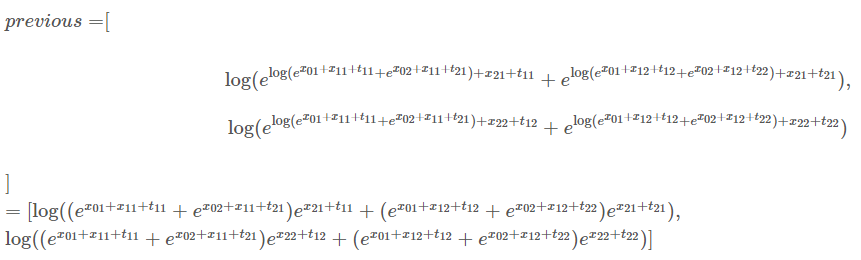就像下一个迭代描述的一样，我们使用新的previous中的元素来计算total score：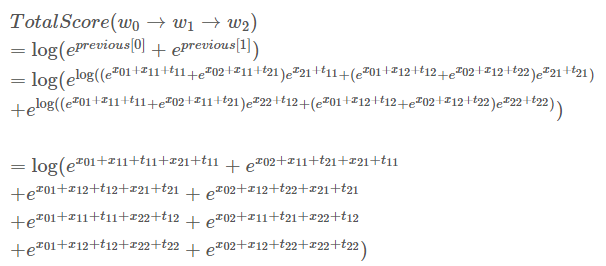恭喜！我们达到了目标，，我们的toy句子有三个单词，label set有两个label，所以一共应该有8种可能的label path。在你享用一杯咖啡或一块甜蛋糕休息之前，请允许我说几句话。虽然你发现这个过程相当复杂，但是实现这个算法要容易得多。使用计算机的优点之一是可以完成一些重复性的工作。现在你可以自己实现CRF损失函数，并开始训练自己的模型。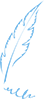—END—英文原文：https://createmomo.github.io/2017/09/23/CRF_Layer_on_the_Top_of_BiLSTM_2交流学习，进群备注：昵称-学校（公司）-方向，进入DL&NLP交流群。方向有很多：机器学习、深度学习，python，情感分析、意见挖掘、句法分析、机器翻译、人机对话、知识图谱、语音识别等。展开全文• 常用的文本识别框架为CNN+BiLSTM+CTC，和CNN+BiLSTM+Attention。经过CNN+BiLSTM将三维图像提取特征，得到2维的特征序列（T*C），然后通过CTC或Attention将特征序列转化为预测结果。 给定一张来自于训练集Q的图像I，...
• 使用pytorch搭建LSTM网络模型并训练的时候，将数据、损失函数和网络模型都放在了显卡上，但是速度仍旧慢的一B。看了看GPU利用率特别低，CPU占用率也不是很高，无法判断到底是什么因素导致训练如此之慢。任务管理器的...pytorch
• 书写LSTM目标函数的时候prediction的tensor和target的tensor 需要计算损失函数，反向传播，为了判断是否可以采用该输入方式的维度利用MSELoss来计算,故验证 MSELoss 计算目标的均方根误差 代码如下 import torch ...pytorch 机器学习
• 这是前向学习的内容，我们知道深度学习需要定义损失函数来进行反向传播，更新梯度。对于序列标注的模型，如何定义损失函数呢？ 我们可以使用CRF损失函数学习模型参数。在这里维特比解码要解决的问题是对于一个新的...自然语言处理
• 这是用LSTM预测未来时刻数据的图片，损失函数采用的是均方误差，为什么得到的训练集和测试集的图片损失函数是上下波动的，波动的幅度还不小，有谁知道原因吗@TOC 欢迎使用Markdown编辑器 你好！ 这是你第一次使用 ...
• 上一节已经实现了LSTM网络的搭建，这一节将实现交叉熵损失函数的搭建和运用，实现对物体的分类。 代码下载地址：xhpxiaohaipeng/xhp_flow_frame 一、softmax+交叉熵损失函数代码实现的原理 1.在经过交叉熵损失函数...深度学习 python
• 深度学习笔记(三)：激活函数和损失函数 深度学习笔记：优化方法总结(BGD,SGD,Momentum,AdaGrad,RMSProp,Adam) 深度学习笔记(四)：循环神经网络的概念，结构和代码注释 深度学习笔记(五)：LSTM 深度学习...
• 《Python深度学习》第三章神经网络入门部分内容，有关神经网络的层和损失函数选取。一、层的选取 1.2D张量，选取全连接层，也叫密集连接层，Keras中对应Dense，例如像神经网络中加入一个512输入，32输出的Dense层 ...神经网络 深度学习 机器学习 人工智能 tensorflow
• 深度学习笔记(一)：logistic分类 深度学习笔记(二)：简单神经网络，后向传播算法及实现 深度学习笔记(三)：激活函数和损失函数 深度学习笔记(四)：优化方法总结(BGD,SGD,Momentum,AdaGrad,RMSProp,Adam) 深度...深度学习 机器学习
• LSTM特性, CNN特性, 损失函数, paper, 项目 ...软件 激活函数: -> sigmod: 硬饱和性, y(0,1), 斜率趋于0;-> tanh: 软饱和性, y(-1,1), 虽然输出均值为0， 可以更快收敛, 但斜率依然会趋于0;-> relu: 当x&...
• 主要的内容有lstm+ctc具体的输入输出，以及TF中的CTC和百度开源的warpCTC在具体使用中的区别。 正文 输入输出 因为我最后要最小化的目标函数就是ctc_loss，所以下面就从如何构造输入输出说起。 tf.nn.ctc_loss ...CTCLoss
• 去掉自定义函数的axis=-1 def rmse(y_true, y_pred): return backend.sqrt(backend.mean(backend.square(y_pred - y_true))) model_lstm.compile(optimizer="adam", loss=rmse, metrics=[rmse])
• [TOC] 关于深度学习的一些理解的参考链接： ... 一、 基础知识 ...1）激活函数 ...sigmoid和tanh函数的导数都是原函数函数。这样，我们一旦计算原函数的值，就可以用它来...1）LSTM t.manual_seed(1000) inp...
• <div><p>项目挺好的，... 能询问一下您用的是什么损失函数吗 (有没有用到lstm、drop out、regularization什么的)，谢谢！</p><p>该提问来源于开源项目：chineseocr/darknet-ocr</p></div>
• 遗憾的是其只输出了每次训练后的损失函数值，并没有可视化训练过程中损失函数的变化。 **题主想得到原文中所示的最优损失函数的下降曲线** ![图片说明]...神经网络 深度学习 python github
• ## LSTM训练无法收敛

千次阅读 2019-03-12 09:44:14
LSTM不收敛问题 ...使用了三层的LSTM,将走的数据当作输入，跑的数据当做标签，将网络输出的结果和我的标签数据进行对比（使用了MSE损失函数），然后反向传播改进网络。 我的问题 网络不收敛，损失函数下降...人工智能 深度学习
• <p><a href="https://www.cnblogs.com/pinard/p/6519110.html">https://www.cnblogs.com/pinard/p/6519110.html</a></p> <p>lstm反向传播中的&#...为什么t时刻的损失函数对于t时刻的加t时刻之后的损失函数？</p>人工智能
• 多输入和多输出模型：使用函数式模型的一个典型场景是...这个模型的损失函数将由两部分组成，辅助的损失函数评估仅仅基于新闻本身做出预测的情况，主损失函数评估基于新闻和额外信息的预测的情况，即使来自主损失函数keras 深度学习 实例
• cell的原理，主要讲述lstm中cell里面的三个门，输入门，遗忘门，输出门，详细介绍如下：http://blog.csdn.net/u014595019/article/details/52605693主要讲解lstm输入的数据格式，...损失函数使用交叉熵，跟cnn类似。TensorFlow
• 多输入和多输出模型：使用函数式模型的一个典型场景...这个模型的损失函数将由两部分组成，辅助的损失函数评估仅仅基于新闻本身做出预测的情况，主损失函数评估基于新闻和额外信息的预测的情况，即使来自主损失函数......